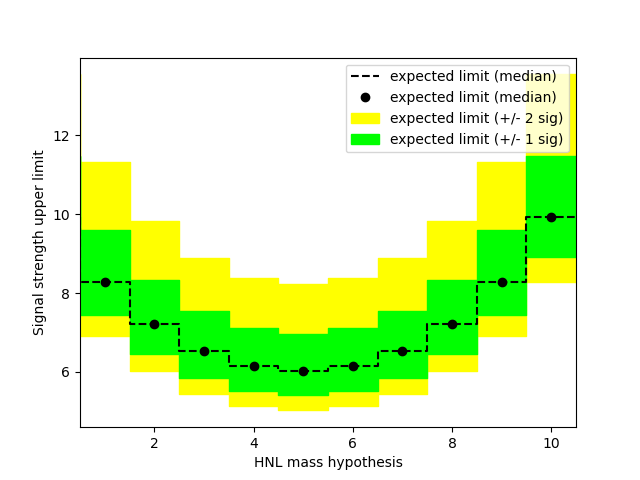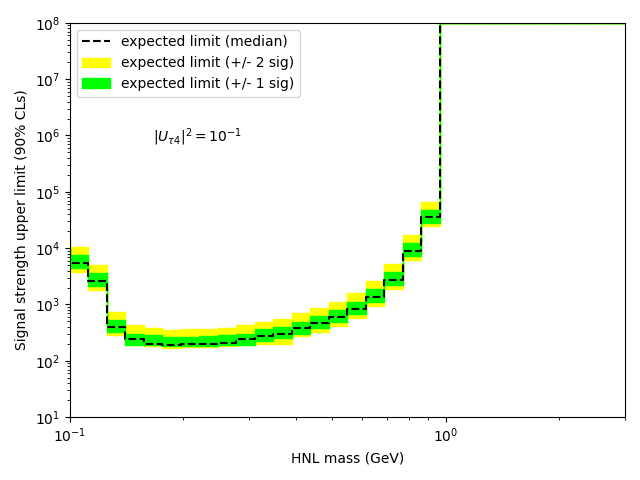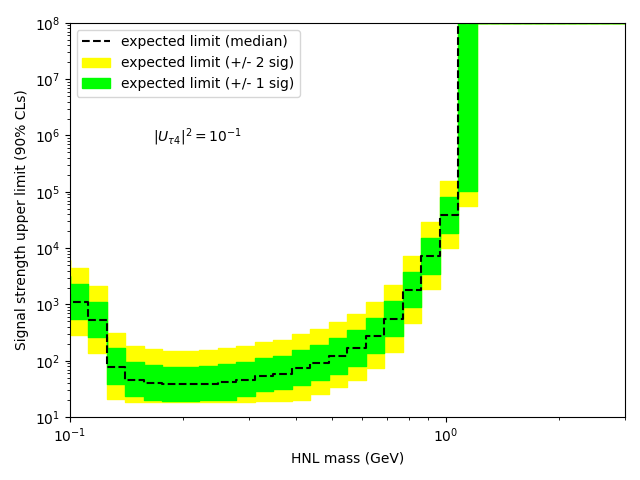# Upper limits as a function of signal parameter

Dear all,

I am trying to compute the upper limit at 95% CLs on the number of signal events as a function of a signal parameter (here the mass of a particle called HNL). I have a certain PDF for the signal that I add above the (constant) PDF for the BG, as is shown on the following plot:

So these are my signal and BG models. Now, if I compute the asymptotic expected upper limit, I get the following scan plot as a function of “nsig”:

My question is: how do I get the upper limit (and corresponding +/- 1 and 2 sigma bands) as a function of the HNL mass? I don’t understand what this plot tells me, why do I only get the p-value as a function of a single scalar (nsig) and not a binned histogram of the HNL mass?

Thanks!

My question is: how do I get the upper limit (and corresponding +/- 1 and 2 sigma bands) as a function of the HNL mass?

Looks like you made your plot by adapting the StandardHypoTestInvDemo. You see your expected and observed upper limits are where the p-value is 0.05, as indicated by the red line. I assume your Brazilian plot was made with the mass fixed to some specific value, right? To get the limit as a function of the mass, you have to run the StandardHypoTestInvDemo multiple times with different values for the mass, as also explained in this forum post.

I don’t understand what this plot tells me, why do I only get the p-value as a function of a single scalar (nsig) and not a binned histogram of the HNL mass?

I can’t see your code, but most likely you have done the plot fixing the HNL mass to some specific value. The plot shows the p-value for your test statistic (should be profile Likelihood one sided) computed with the asymptotic formulae as explained in this reference (page 36 in the pdf, the p-value is alpha in the formula). In particular, the plot tells you that your median expected upper limit is roughly 8 times the standard model prediction (ready by eye from the plot, the actual numeric values can be found in the standard output text), and the distribution of the upper limit is given by the uncertainty band. As explained above, to get limits as a function of the HNL mass you have to run this procedure multiple times for different values of the mass.

By the way, you’re saying “these are my signal and BG models”, but I only see a single histogram. What’s that? Signal, background, or both?

I hope my answer helps you, if you still have further questions or if something is unclear, don’t hesitate to ask!

Cheers,
Jonas

Hello Jonas,

Thanks a lot for your answer, this is very clear! I think I went a little too far when I said “I don’t understand why this plot is giving me”. I should just have said “I don’t understand why it gets computed for a single mass only and not for the mass points corresponding to the mass histogram”. The fact that you say this is for a single mass that must have been specified somewhere in my code is both reassuring (because it corresponds to the way I understand this) and confusing (because I didn’t purposely fixed the mass). I attach my code here (I would recommend starting at line 190), maybe you can identify where the mass is fixed and how I can change that to scan the limit as a function of the mass? Is it at line 312 where the “parameter of interest (poi)” is set to 0?

Regarding the signal and BG models, the histogram you see is the sum of both. The low mass bump corresponds to the signal, added on top of a constant BG (that dominates at high masses).

Thanks!
compute_limits.py (13.5 KB)
.

Hi, thanks for sharing the script!

I see that your dataset has the “hnlMass” as the observable. So when we talk about HNL mass here, we have to distinguish two different variables. On one side there is the observed mass, on the other side there is the HNL mass that you set in you model right. However, I don’t wee the HNL mass model parameter in your script.

I took a look at the lines 240 to 250 in your script, where the signal model is defined:

``````obs = ROOT.RooArgSet()
hist_temp = ROOT.TH1D("hist","hist",30,0.1,3.)
bins = np.linspace(0.1,3.,31)
array_binned, _ = np.histogram(data,bins,weights=data_weights)
hist = array2hist(array_binned,hist_temp)
dataHist = ROOT.RooDataHist("hist","hist",obs,hist)
sigPDF = ROOT.RooHistPdf("histpdf","histpdf",hnlMass,dataHist,0)
nsig = ROOT.RooRealVar("nsig", "nsig", 0, -10, len((data)))
sig_norm = ROOT.RooExtendPdf("sig_norm", "sig_norm", sigPDF, nsig)
``````

Put in words, you are creating the template from the signal model from “data”, which is:

``````data = np.concatenate((signal,bg))
``````

So one thing that’s probably wrong is that you consider the background in the signal template. The other problem is that you take the shape from the signal template from the data that you fit the template to. So something is fishy there: you double count your background and you fit the data to a template defined by the data.

My question is: how do I get the upper limit (and corresponding +/- 1 and 2 sigma bands) as a function of the HNL mass?

…I thought that what you had was a model parametrized in the HNL mass, so you can get an upper limit for each probed mass. But this would also mean that you need a separate signal template for each mass value, possibly interpolating between them. I don’t understand how you can’t have a parametrized signal model in your script.

So basically you are fixing the mass to the mass observed in the data, but I don’t think that this is what you ultimately want to doI hope this gives you some ideas on what you need to adjust in your fit!

Cheers,
Jonas

Hello Jonas,

Ok you’re right, this is messy. Let me try to clearly state what I’m trying to do. I have a certain mass distribution for my signal, and another one for the background (which happens to be constant since the bg does not depend on signal parameters). These give me the expected number of events for the signal and the bg as a function of the signal parameter (here the HNL mass). My understanding is that these distributions define my signal and bg models, isn’t that true/enough? What I want to do is to compute the expected upper limit on the number of events corresponding to these distributions, i.e. have an upper limit (and +/- 1/2 sigma bands) for each bin of the histogram. I understand this expected upper limit to be the upper limit computed assuming the observed data is set to the null hypothesis, i.e. is equal to the background only. Now, the mass distribution for my signal does not follow any known distribution (Gauss, Breit-Wigner, Landau, etc.), so instead of fitting my signal distribution to get a smooth PDF I want to have the signal model follow the signal histogram (that’s why I use sigPDF = ROOT.RooHistPdf()). I now realize I am summing a histogram PDF (for my signal) and a continuous PDF (for my bg), maybe that’s also not very proper?

Hi David,

to address the easy concern first:

I now realize I am summing a histogram PDF (for my signal) and a continuous PDF (for my bg), maybe that’s also not very proper?

That’s fine, with RooFit you can add any pdfs together, also continuous pdfs with binned pdfs.

Okay, so then do I understand correctly that what you do is actually not a search for a signal parametrized in mass, but you specifically search for the signal with the single distribution specified in your sigPDF?

But if you’re search is not parametrized, how does it make sense to have an expected upper limit as a function of the mass (for each bin of the histogram as you say)?

The only other thing that would make sense to me is if you want to consider each bin as a separate counting experiment and get an upper limit for each bin in isolation. This would of course not be something you quote as the final result of your experiment, but sometimes you still want to know these upper limits for individual bins to quantify the sensitivity of each bin. Is this what you want to do?

Sorry the confusion, when you talked about “upper limit as a function of the HNL mass” I automatically thought of parametrized searches like the one leading to the Higgs discoveryCheers,
Jonas

Yes, that’s it! I want to estimate the sensitivity for each bin, like each bin is a separate counting experiment. This is because the HNL mass is here a parameter and not an observable (that’s why the background is constant as a function of this parameter!). Thanks for properly phrase this. It differs from a standard “bump hunt” as a function of the invariant mass for instance, because here the signal parameter is different for each bin, while in a classic bump hunt you fix the signal mass (the parameter) and scan the invariant mass (the observable). Ok, so now, what should I change for this to make sense?

I attach a simplified version of the code that shows what I think I should be doing. I’ve run it and I don’t think it’s quite correct yet, since it gives me similar results whatever the number of signal events.
compute_limits_debug.py (11.0 KB)

okay, thanks for confirming this! Okay, so there is no differential observable in your measurement then. RooStats still needs at least one observable to work properly though.

Maybe what you can do is to create two extended RooUniforms for signal and background, and the expected number of signal events depends depends on your mass parameter that you get from your histogram? You can then do the upper limit extraction for each mass value in your histogram, save the results of the HypoTestInverter and plot them.

I have quickly hacked together a standalone example to explain what I mean:
compute_limits_example.py (3.8 KB)

The output of this example should look like that:It’s just an example, so I guess from the physics point of view it doesn’t make sense that the highest predicted cross section and hence sensitivity is for this arbitrary value of 5.0I hope this code example gives you some inspiration to solve also your problem! In any case, feel free to continue asking questions.

Cheers,
Jonas

Hello Jonas,

Thanks so much for this, it’s super helpful. I have a few questions though:

• Why is there a standard model dependence on the signal parameter in your example (it’s a Gauss distribution)? Shouldn’t it be flat by construction since the SM does not depend on the signal?
• Why do you call the standard model prediction “nsig_sm”? Shouldn’t the SM prediction be the background (bkg) rather than the signal (sig)?
• As a consequence, I don’t get why on lines 34-35, the signal model is defined as the signal strength times nsig_sm, but the background is not simply nsig_sm? If the signal is a certain scale (mu) times the SM expectation (the SM background), why isn’t the background just nsig_sm without the scale?

Sorry I’m still confused by some stuff here…

Hi David,

oh yes you are right, I messed the names up! Everything that has the `_sm` suffix should better be `_bsm`, because it is supposed to the like a search for a BSM signal where the signal yield depends on the mass of the BSM particle. My bad.

By the way, in my example I take the parametrized BSM signal yield from a RooGaussian. In your code you can do that with your RooDataHist, but actually it could also just be a RooRealVar where you set the value directly from this DataFrame you have.

I hope it makes a bit more sense now, otherwise just continue askingCheers,
Jonas

Hi Jonas,

Ok that makes much more sense to me!

Maybe another thing: I would expect the BG yield to be fixed at a certain value (equal for all values of the signal mass), where is this done in your code? Line 35 seems to be saying that the number of BG events can vary between 0 and 100, but my understanding is that the BG is known and should not be varying.

Thanks!

Hi!

Well, the expected number of background events certainly has a certain value, which I set to 20 in my example. This nominal value is then used the the dataset generation, including the generation of the Asimov dataset done internally by the AsymptoticCalculator.

But since the background is also a statistical Poisson process, the number of background events has to be floating in the fit. In other words, the background yield is a nuisance parameter, constrained by a Poisson distribution with mean 20. That’s usually how these fits workCheers,
Jonas

Hello Jonas,

Thank you so much for your help, I think I was able to get what I wanted, see the following plot:The fact that it doesn’t work at large masses is caused by the scanning range of the test statistics that is too small/not optimized (correct me if I’m wrong).

I may have a last question for you: say I want to add a systematic uncertainty on the bg for instance, is it correct to simply move from a uniform pdf for the nbg to a Gaussian, the width of which being the uncertainty? In the code, this would look like (for a 10% uncertainty):

``````#bkg = ROOT.RooUniform("bkg", "bkg", x)
mean_bg = ROOT.RooFit.RooConst(bg)
sigma_bg = ROOT.RooFit.RooConst(0.1*bg)
bkg = ROOT.RooGaussian("bkg", "bkg", x, mean_bg, sigma_bg)
``````

The result I get is the following:We clearly see the larger uncertainty bands. The median also changed (I get a better sensitivity with the uncertainty in), which I would not expect, but I increased the number of scanning points, so it may be the consequence of a better precision?

Also, if I don’t add any systematic uncertainty, what exactly is driving the +/- 1 and 2 sigma bands in the brazilian band? Only the statistics of the event counts for the signal and the bg?

Thanks!

Any idea regarding the last question?Sure! This was followed up on in Including systematic uncertainties to a model.

This topic was automatically closed 14 days after the last reply. New replies are no longer allowed.